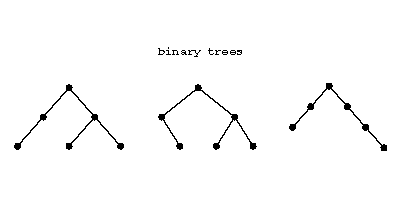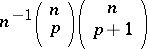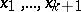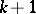# Binary tree

(diff) ← Older revision | Latest revision (diff) | Newer revision → (diff)

A (planar) rooted tree for which every node has a left child, a right child, neither, or both. Three examples are:Figure: b110530a

These three are all different.

The number of binary trees withnodes,left children,right children () isThe numbersare called Runyon numbers or Narayama numbers.

A complete binary tree is one in which every node has both left and right children or is a leaf (i.e., has no children). E.g., there are two complete binary trees with five nodes:Figure: b110530b

A complete binary tree has an odd number of nodes, say, and then the number of leaves is. Label theleaves from left to right with symbols. Then the various complete binary trees with theirleaves labelled in this order precisely correspond to all the different ways of putting brackets in the word, each way corresponding to a computation of the product by successive multiplications of precisely two factors each time. The number of ways of doing this, and hence the number of binary trees withnodes, is the Catalan numberThe problem of all such bracketings of a product (of numbers) was considered by E. Catalan in 1838 [a1].

The correspondence between complete binary trees and (complete) bracketings gives a bijection between complete binary trees with leaves labelled with elements from a setand the free magma on.

How to Cite This Entry:
Binary tree. Encyclopedia of Mathematics. URL: http://encyclopediaofmath.org/index.php?title=Binary_tree&oldid=18931
This article was adapted from an original article by M. Hazewinkel (originator), which appeared in Encyclopedia of Mathematics - ISBN 1402006098. See original article